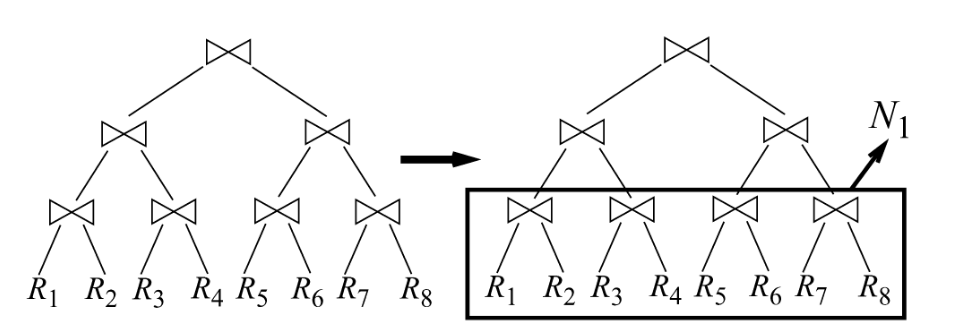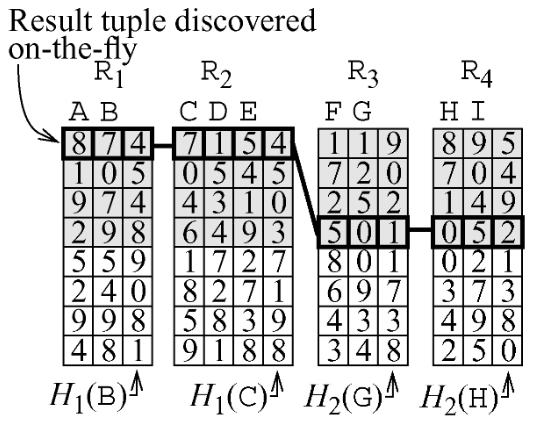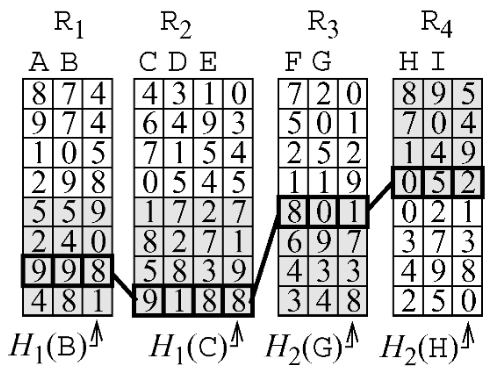CSE-4/562 Spring 2019 - OLA and AQP

### OLA and AQP

#### CSE-4/562 Spring 2019

March 4, 2019

What is the best, correct technique for task X, when Y is true?

1. How do you define Correct and Best?
2. What correct alternatives are available?
3. How do you find the best available alternative

So Far: Correct = The original RA for a query.

Only queries guaranteed to produce identical results are correct

What happens when Correct allows for a margin of error?

• Aggregate query with some margin of error.
• ORDER BY LIMIT (Top-K) with some margin of error.
• LIMIT without ORDER BY (Any-K).

Sacrifice a little accuracy for a lot of speed

### Approximate Query Processing


SELECT SUM(A) FROM R


Naively, you need to see all values of R.A

### Online Aggregation

$Avg(3,6,10,9,1,3,9,7,9,4,7,9,2,1,2,4,10,8,9,7) = 6$

$Avg(3,6,10,9,1) = 5.8$ $\approx 6$

$Sum\left(\frac{k}{N} Samples\right) \cdot \frac{N}{k} \approx Sum(*)$

Sampling lets you approximate aggregate values with orders of magnitude less data.

With $n$ tuples sampled uniformly with replacement

 $|AVG(samples) - AVG(real)|$ The absolute error $P(|AVG(samples) - AVG(real)| \geq \epsilon)$ Its probability of exceeding error threshold $\epsilon$ $P(|AVG(samples) - AVG(real)| \geq \epsilon) \leq 2e^{\frac{2n\epsilon^2}{(max(real) - min(real))^2}}$ ... is below a threshold based on $\epsilon$, $n$, and the min/max value.

"Hoeffding's Bound"

### Bootstrapping

Idea 1: Generate a bunch of samples of the same size and see how they're distributed.

The resulting histogram models the distribution of samples.

Problem: Generating samples is expensive!

Idea 2: Generate one sample, then resample to see how its distributed

The resulting histogram still models (in expectation) the distribution of samples.

### Error Bounds

SUM, COUNT, AVG (sampling with replacement)
Hoeffding's Bound
Chernoff's Bound
SUM, COUNT, AVG (sampling without replacement)
Serfling's Bound
Any Other Aggregate
BootstrappingKeep adding samples until you reach a target accuracy

Keep adding samples until you run out of time

### Generating Samples

Sampling From Disk
Random seeks are slow
Sampling For Group-By or Selections
Low-frequency events don't get sampled
Sampling From Joins

### Sampling From Disk

Idea 1: Pick Randomly!


for i from 1 to num_samples:
sample_id = random(0, num_records)
samples += [ table.where( rowid = sample_id ) ]Problem: Random scans are EXPENSIVE.

Idea 2: Assume data already randomized!

Pick a random start record and read sequentially from there.Problem: Sequential records are almost never IID.

Idea 3: Prebuild samples!

Shuffle data into fixed size sample buckets (e.g., BlinkDB).

### Sampling From Group-By

EmployeeCitySalary
AliceNYC$120k BobNYC$110k
CarolNYC$115k DaveBuffalo$80k

SELECT City, AVG(Salary) FROM NYS_Salaries;


Problem: Most data is about NYC. With $N$ samples taken uniformly, margins of error for other cities are much bigger

### Stratified Sampling

Generate $\frac{N}{\texttt{COUNT}(\texttt{DISTINCT} City)}$ samples for each group

Use $\texttt{COUNT}(\texttt{DISTINCT} City)$ instead of $\texttt{COUNT}(*)i$ as the total group size

### Index Striding

Idea 2: Pre-generate sample buckets across a range of different strata (e.g., BlinkDB).

### Sampling from Joins

Assume: $\texttt{UNIQ}(A, R) = \texttt{UNIQ}(A, S) = N$

It takes $O(\sqrt{N})$ samples from both $R$ and $S$
to get even one match.

### Weighted Joins

Exploit Foregin Keys
Sample from the referencing table, join with full reference tables
Stratified Sampling
For many-many joins, stratify on the join attribute(s)

### Convergent Joins

Ripple Join
Incrementally increase the sample size
Turbo DBO Join